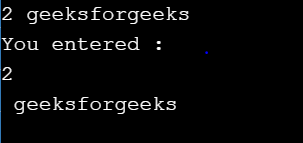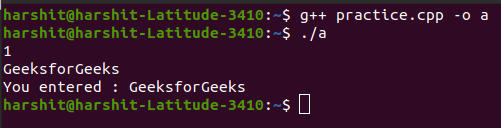• Last Updated : 04 May, 2021

Program 1:

Below is the program that inputs a string with spaces just after taken an input of an integer

## C++

 `// C++ program that inputs a string ` `// with spaces just after taken an ` `// input of an integer ` ` `  `#include ` `#include ` `using` `namespace` `std; ` ` `  `// Driver Code ` `int` `main() ` `{ ` `    ``int` `t = 0; ` `    ``cin >> t; ` ` `  `    ``string s; ` ` `  `    ``// Taking input with spaces ` `    ``getline(cin, s); ` ` `  `    ``cout << ``"You entered : "` `         ``<< s << ``"\n"``; ` ` `  `    ``return` `0; ` `} `

Output:Explanation:

• In the above code, the string variable S is not able to store our input.
• The reason for this is that, when entering the integer T, and pressed enter, the newline character (\n) was not stored in the integer variable T.
• Instead, this newline character was stored in the upcoming string variable S.
• Hence, when displaying string S, it gives output as blank spaces. This is true even if the data type preceding the string is not int. It can be any data type besides int.
• As soon as enter is pressed, the newline character will get stored into the string which is inputting.

Program 2: In C++, the ignore() function accounts for nullifying the extra newline character which is generated by pressing “enter”. Below is the C++ program illustrating the use of the function ignore():

## C++

 `// C++ program to illustrate the use ` `// of the function ignore() ` ` `  `#include ` `#include ` `using` `namespace` `std; ` ` `  `// Driver Code ` `int` `main() ` `{ ` `    ``int` `t = 0; ` `    ``cin >> t; ` ` `  `    ``// Adding the ignore() ` `    ``cin.ignore(); ` ` `  `    ``string s; ` `    ``getline(cin, s); ` ` `  `    ``cout << ``"You entered : "` `         ``<< s << ``"\n"``; ` ` `  `    ``return` `0; ` `} `

Output:My Personal Notes arrow_drop_up
Recommended Articles
Page :